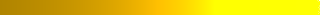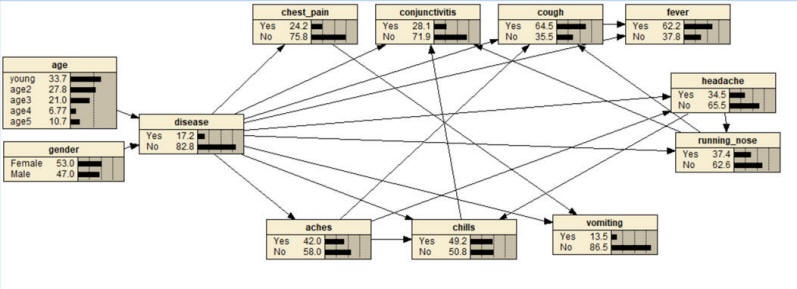﻿ Temporal Analysis# Temporal Analysis

• Construction of network model from regressions (use instructor's last name as password) Read►
• Temporal analysis
• Chain of Regressions
• Python code for LASSO Logistic Regression with interaction terms ChatGPT►
• Learning network structure from regressions Slides►
• Parameters of Network model
• Learning joint distribution of variables from regressions Slides►
• Python and R code for LASSO regressions, temporal analysis, R-squared calculations, and other topics Zip►

## Assignment

For this assignment you can use any statistical software.  p>

Question 1:  Classify COVID-19 based on its symptoms.

1. Describe the order of occurrence of the variables
• Assume that age, and gender occur at birth.  Assume that vaccination information occurs before onset of symptoms.  Assume home tests occurs after onset of symptoms.  Assume that laboratory PCR test occurs after home test.
• Establish the order with which symptoms occur
• Count for each pair of symptoms, the number of times one symptom occurs before another.  Use column AK in the database to identify if one symptom has occurred before another.
• Use the pairwise count of one symptom occurring before another to establish a sequence of occurrence of symptoms. Only prior symptoms can be independent variables in analysis of later symptoms.
2. Create a Causal Network for clusters of symptoms of COVID-19
• Create the structure of the network:
• Using LASSO, regress the PCR test results on all variables and pairwise or triple cluster of variables that precede it.
• Using LASSO, regress each variable that is a direct predictor of PCR test results on all preceding variables. In these regressions, statistically significant variables are parents in the Markov blanket of the regression response variable.
• Draw the network using Netica.
• Estimate the parameters of the network
• Using the LASSO regression, calculate the predicted value for all combinations of the parents in the Markov blanket of the regression's response variables. Enter this information into Netica Tables.
3. What is the probability of COVID for a patient less than 30, female, with runny nose, muscle aches, and with unknown fever status. What is the same probability if we knew that the patient does not have COVID.
4. What are the parents in the Markov blanket of fever?

The following resources may be helpful:

Question 2: Using the data provided and Netica software, construct a network model of COVID-19, Influenza, and other upper respiratory infections symptoms. Create a node at the center with three levels.

• First, LASSO regress the disease variable on all symptoms, age and gender. This regression will identify variables in the Markov blanket of the disease.
• Second, identify parents and children in the Markov Blanket of the disease variable.  Symptoms, by definition, occur after the disease and demographic variables occur prior to the disease.  Using Netica software, draw an arrow from the disease variable to symptoms that were statistically significant in the LASSO regression.  Similarly, draw an arrow from demographic variables to the disease, if the demographic variable was a significant predictor in the LASSO regression.
• Third, use each symptom that is statistically significant in first step as a response variable for a new LASSO regression.  The independent variables in these regressions are the disease variable, other symptoms, and gender. Using Netica, draw an arrow from the variables that are statistically significant to the symptom used as response variable. Repeat for other symptoms as response variable. Here is a sample of the results of these regressions.  Note that findings above 0.15 or below -0.15 are listed, the choice of 0.15 is arbitrary focus on large magnitude associations. The relationships from age and gender to the symptoms are not listed as all were not significant. Relationships that can create a cycle are crossed out.

 Aches Chest Pain Chills Red Eye Cough Diarrhea Fatigue Fever Head- ache Nausea Runny Nose Short Breath Vomit Wheeze Aches 0.24 0.17 0.37 0.22 Chest Pain 0.17 Chills 0.18 0.41 0.16 Red Eye 0.40 Cough 0.27 0.17 Diarrhea Fatigue 0.36 0.18 0.18 Fever 0.21 Headache 0.21 0.21 0.18 0.16 Nausea 0.54 Runny Nose -0.16 0.21 0.15 Short Breath 0.28 0.36 Vomit 0.15 0.69 Wheeze 0.21 0.38

• Fourth, enable Netica software to learn the parameters of the model or generate the joint distribution of the variables from regression equations
• Fifth, report the probability of COVID-19 in a patient with fever, cough and runny nose and unknown other symptoms.  Set these three nodes to symptom being present and read the network probability for COVID-19.

Resources for question 2:Question 3: This problem shows how temporal analysis can be done based on pairwise information on occurrence of variables.  The following data shows the percent of people for which the symptom listed in the row occurs before the symptom listed in the column.  Identify which symptom occurs first.  Which symptom occurs last.  Which two symptoms occur closest to each other.

Resources for Question 3

Question 4: In your All of Us project, conduct a temporal analysis counting the number of people for whom one condition precedes another.  Include the code for conducting such analysis in response to this question.